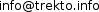# Planetary System Formation 2

## N-body simulation

 Arithmetic precision: 64 bits Number of objects: 400 Number of objects at the end: 10 Number of cycles: 17 780 000 Seconds per cycle: 5 Simulation time: ~ 2.8 years Objects are placed in a rectangle with diagonal: 4.2384e+11 m For comparison - Neptune (Solar system) semi-major axis: 4.503443661e+12 m Lightest object mass: 1.0224e+22 kg Initial heaviest object mass: 2.1469e+24 kg Initial average object's mass: 6.0661e+23 kg Heaviest object mass at the end: 2.3249e+26 kg ~ 96% of total Total mass of all objects: 2.4264e+26 kg For comparison - Earth's mass: 5.9736e+24 kg For comparison - Jupiter's mass: 1.8986e+27 kg Total mass of the 8 Solar system planets: 2.66731443e+27 kg Min object's radius: 1.5964e+6 m ~ 1596 km Initial max object's radius: 9.4883e+6 m ~ 9488 km Average object's radius: 4.8424e+6 m ~ 4842 km Heaviest object radius at the end: 4.5226e+7 m = 45 226 km For comparison - Earth's mean radius: 6.371e+6 m = 6371 km For comparison - Jupiter's mean radius: 6.9911e+7 m = 69 911 km Objects density: 600 kg/m3 = 0.6 g/cm3 For comparison - Earth's mean density 5515 kg/m3 = 5.515 g/cm3 For comparison - Jupiter's mean density 1326 kg/m3 = 1.326 g/cm3 Initial min object's speed: 241.02 m/s ~ 868 km/h Initial max object's speed: 4075.8 m/s ~ 14 673 km/h Average object's speed: 2323 m/s ~ 8363 km/h For comparison - Moon average orbital speed: 1022 m/s ~ 3679 km/h For comparison - Earth average orbital speed: 29 780 m/s ~ 107 208 km/h For comparison - Jupiter average orbital speed: 13 070 m/s ~ 47 052 km/h Interacting law: Newton's law of gravity

Data from NASA and Wikipedia.

You can send me your ideas for further simulations at:More simulations

## Коментари

### Публикувай нов коментар

• Адресите на уеб-страници и e-mail адресите автоматично се конвертират в хипервръзки.
• Разрешени HTML tag-ове: <a> <p> <span> <div> <h1> <h2> <h3> <h4> <h5> <h6> <img> <map> <area> <hr> <br> <br /> <ul> <ol> <li> <dl> <dt> <dd> <table> <tr> <td> <em> <b> <u> <i> <strong><font> <del> <ins> <sub> <sup> <quote> <blockquote> <pre> <address> <code> <cite> <embed> <object> <param> <strike> <caption>
• Линиите и параграфите се прекъсват автоматично.
• Mark language-dependent sections with == lc == where lc (or lc-xx) is a language code, other or all.

Повече информация за опциите на форматиране